# Using Text Search & Date Maths in Outgrow's excel builder

In Outgrow, the Excel builder functionality under formula builder offers the ability to understand the text. Users can now search for values in a string such as does the text input answer to Q10 include the following text or show the specific value on the specific date. You can go through this document to learn more about how this can be achieved.

# Achieving text based search

1. Let's Say value in cell A1 is a string. In this case, we can have it as Wrong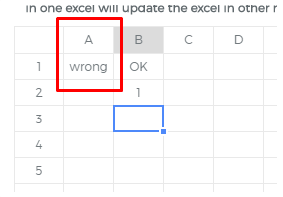2. Now if we try to use a formula in excel builder which will be = IF(A1="wrong", "OK", "Wrong number"), it will output OK, but this output cannot be used in the result. The reason for this is that our formula builder only accepts a numerical value as an output.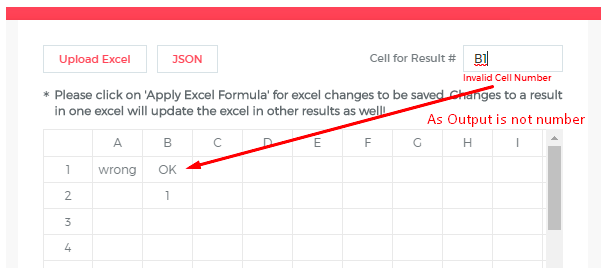3. In case the formula in excel builder is = IF(A1="wrong", 1, 2), it will output 1 as the value, and this value can be used as a result, which can be used in Conditional Messages as well.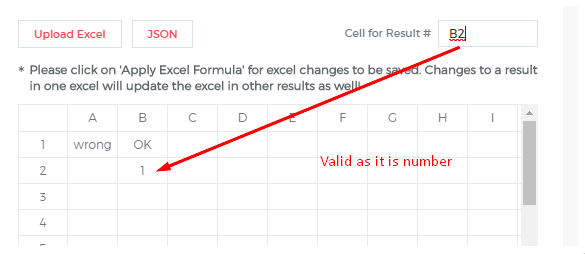# Achieving displaying of a specific value when a specific date is selected

1. Let's say value in cell A1 is in date format and is 02-11-2019 (specified in MM-DD-YYYY format). Now, in this case, the user wants that the date should be converted to a numerical mapping so in this case the user can enter the formula in B7 which is =DATEVALUE(A7). DATEVALUE will convert the date to numerical mapping.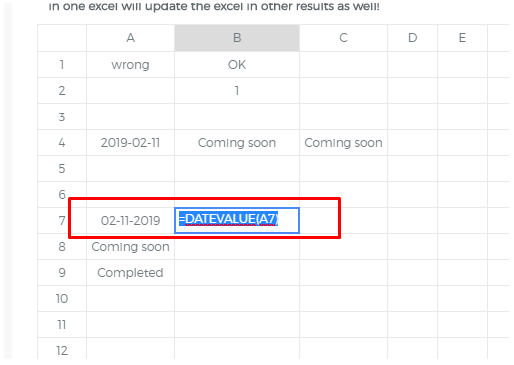2. Now there are 2 different formulas that can be used depending on whether when a date is specified the user wants to display Completed or Coming soon. For now let's consider that in A8 the user wants to display Coming Soon. So in A8 the formula that needs to be added is =IF(B7<DATEVALUE("02-10-2019"), "Completed", "Coming soon"). This will output Coming Soon.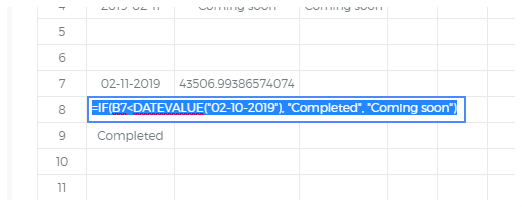Same way in case the user wants to display Completed as the output, the formula that we need to add in A9 Formula is =IF(B7<DATEVALUE("02-12-2019"), "Completed", "Coming soon").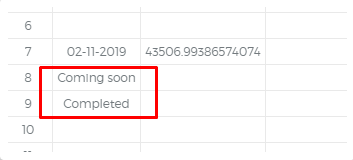NOTE: You need to make sure that the date format that you use across all the formulas should be the same. You need to use MM-DD-YYYY across all the formulas.

Feel free to use our chat tool on the bottom right or reach out to us at [email protected] in case you have any questions or feedback and our team will be glad to assist you further.